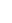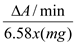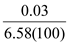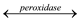# Modern Experimental Biochemistry

Chemistry

## Quiz 12 : Activity and Thermal Stability of Gel-Immobilized PeroxidaseStudy FlashcardsLooking for Biochemistry Homework Help?

## Quiz 12 :Activity and Thermal Stability of Gel-Immobilized Peroxidase

Question TypeThe data below were collected by students completing this lab. From their data, calculate the units of enzyme activity per mg of gel. Amount of gel = 100 mg ∆ A /min = 0.03 per minute
Free
Essay

In this problem, we are asked to calculate the specific activity of the enzyme from data collected by other students who completed the experiment. Their data for ?A/min is 0.03, and the number of milligrams of gel they used was 100mg.
The formula to calculate specific activity is as follows:specific activity =Using this data, we calculate the specific activity as follows:Or, 0.00005 units/mg.

Tags
Choose question tagThe breakdown of hydrogen peroxide catalyzed by peroxidase is an oxidation-reduction reaction. Study Equation E12.1 and answer the following questions. (a) What is the reducing agent? (b) Is H2 O2 oxidized or reduced (c) What is the cofactor for peroxidase?
Free
Essay

In this problem, we are asked several questions about the following reaction:
H2 O2 + AH22H2 O + A
First, we must state the reducing agent. Second, we must state if hydrogen peroxide (H2 O2 ) is oxidized or reduced, and finally, what is the cofactor for peroxidase.
a)The reducing agent, of the material that is oxidized, is AH2.
b)The peroxide is reduced.
c)Peroxidase uses heme molecules as a cofactor.

Tags
Choose question tagHow would you change the experimental conditions in order to trap an enzyme that has a molecular weight over 1 million? Horseradish peroxidase has a molecular weight of 40,000.
Free
Essay

In this problem, we are asked how we would immobilize a much larger enzyme than the horseradish peroxidase. The peroxidase has a molecular weight of 40 kilo-Daltons, and the theoretical enzyme in question is 1000 kilo-Daltons.
Polyacrylamide gel will not work well for immobilizing such a large enzyme; the pore sizes of even highly uncross-linked gels are too small to hold onto such a large enzyme. We would need a different method, such as adsorption onto an insoluble polymer support system (like calcium alginate beads). The remainder of the experiment would be largely similar.

Tags
Choose question tagWhat is the purpose of filtering the assay tube in part B before reading A 510 ?
Essay
Tags
Choose question tagHow could you determine if the gel is "leaking" peroxidase? What experimental modifications could be made to prevent this?
Essay
Tags
Choose question tagWhat should be the shape of a plot of ∆ A /min (immobilized enzyme activity) vs. milligrams of gel? Explain.
Essay
Tags
Choose question tagWhat are some of the benefits of using immobilized enzymes in a commercial process?
Essay
Tags
Choose question tagWhat is the purpose of washing the acrylamide gel several times with water as described in the experimental section, part A? How could you experimentally determine when washing is complete?
Essay
Tags
Choose question tagDesign an experiment using the immobilized peroxidase to calculate the Michaelis constant, K M , and the maximum velocity, V max.
Essay